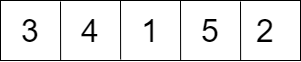# Bubble Sort

The most basic and easy to implement sorting technique is Bubble Sort. It works by simply comparing each and every element and gets its name from the way in which the smaller elements bubble to the starting of the array.

## Understanding Bubble Sort

Every possible pair is compared and swapping is performed if required, that is, if there are ‘n’ elements then n*n comparisons will be done, though it’s time-consuming, it places elements in their correct indexes in the end.

## Algorithm for Bubble Sort

• for i = 0 to length of Array
• for j = 0 to length of Array - 1
• if Array[j] is greater than Array[j+1]
• then, Swap vaules at Array[j] and Array[j+1]
• End inner for loop
• End outer for loop

## Bubble Sort Example

Consider an Array : `5 3 4 1 2`Length of Array = `5`

There will be 5 iterations of the outer loop

### Iteration Number 1

``````j = 0
5 will be compared with 3
Since 5 > 3
Swapping will be done``````

Array after swapping will look like:``````j = 1
Now 5 will be compared with 4
Since 5 > 4
Swapping will be done``````

Array after swapping:``````j = 2
5 will be compared with 1
Since 5 > 1
Swapping will be done``````

Array after swapping:``````j = 3
5 will be compared with 2
Since 5 > 1
Swapping will be done``````

Array after swapping:### Iteration Number 2

``````j = 0
3 will be compared 4
Since 3 < 4
Nothing will be done

j = 1
4 will be compared with 1
Since 4 > 1
Swapping will be done``````

Array after swapping:``````j = 2
4 will be compared with 2
Since 4 > 2
Swapping will be done``````

Array after swapping:``````j = 3
4 will be compared with 5
Since 4 < 5
Nothing will be done``````

### Iteration Number 3

``````j = 0
3 will be compared with 1
Since 3 > 1
Swapping will be done``````

Array after swapping:``````j = 1
3 will be compared with 2
Since 3 > 2
Swapping will be done``````

Array after swapping:Now we can see that Array is sorted, although more comparisons will be done, no swapping will be done hereafter.

Let’s look at the Java code for the same.

## Code for Bubble Sort

``````import java.util.Arrays;

public class Sorting {
void bubbleSort(int arr[]) {
int lengthOfArray = arr.length;
int temp; // Temporary variable for swapping
for (int i = 0; i < lengthOfArray; i++) {
for (int j = 0; j < lengthOfArray - 1; j++) {
if (arr[j] > arr[j + 1]) {
temp = arr[j + 1];
arr[j + 1] = arr[j];
arr[j] = temp;
}
}
}
}

public static void main(String[] args) {
Sorting sort = new Sorting(); // creating object of class Sorting
int[] arr = { 5, 2, 6, 1, 3 };
sort.bubbleSort(arr); // method call
System.out.println("Array after applying bubble sort : " + Arrays.toString(arr));
}
}``````

## Performance

CaseRuntime
BestO(n^2)
AverageO(n^2)
WorstO(n^2)
Space complexityO(1)

Bubble sort might be very easy to implement but it has a complexity of n^2 in all three cases.

We can improve the best case of bubble sort to O(n) by using a flag which will indicate if the array is already sorted or not.